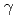※ 半角英数字
年 ～
年

# Consistency check of experimental data using evaluated photonuclear data

## 評価済光核データに基づく測定データの整合性確認

Evaluation of photonuclear data has been performed by relying on experimental data. Recently, Varlamov et al. introduced neutron-multiplicity transition functions to investigate the validity of partial photoneutron cross sections. The neutron-multiplicity transition functions were defined as a ratio of partial photoneutron cross section to the neutron yield cross section. These functions take values smaller than 1 or 0.5 if the photon energy is below or above the threshold energy of (,2n) reaction channel. They pointed out that there is violation of measured cross sections of (,1nx) and (,2nx) reactions. We applied this methodology and checked whether the measured data can be used to evaluate the photoneutron cross sections. In order to do this, the photon-induced reaction cross sections were calculated by using a nuclear reaction calculation code CCONE, and then, the neutron-multiplicity transition functions were derived. The covariance estimate was simultaneously performed with the KALMAN code, considering the ambiguity of adopted model parameters. The calculated results for the partial photoneutron and neutron yield cross sections were compared with the experimental data. It is found that the whole experimental data are not necessarily useless for evaluation, though some of them violate the criteria of neutron-multiplicity transition functions.